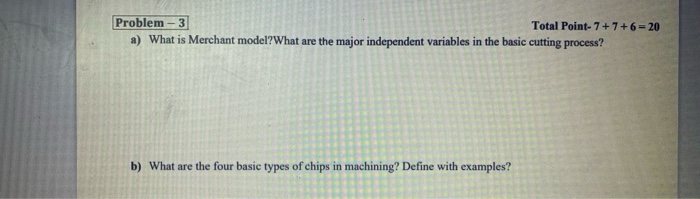# Question Problem - 3 Total Point- 7 + 7 + 6 = 20 a) What is Merchant model?What are the major independent variables in the basic cutting process? b) What are the four basic types of chips in machining? Define with examples?0A1AP6 The Asker · Mechanical EngineeringTranscribed Image Text: Problem - 3 Total Point- 7 + 7 + 6 = 20 a) What is Merchant model?What are the major independent variables in the basic cutting process? b) What are the four basic types of chips in machining? Define with examples?
More
Transcribed Image Text: Problem - 3 Total Point- 7 + 7 + 6 = 20 a) What is Merchant model?What are the major independent variables in the basic cutting process? b) What are the four basic types of chips in machining? Define with examples?